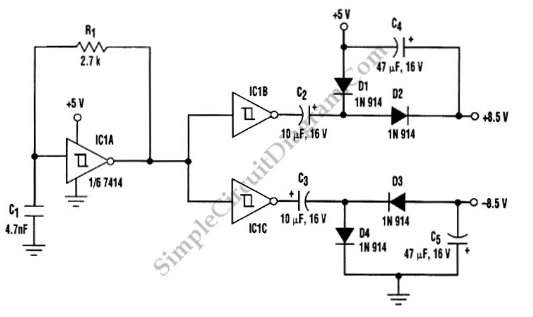# Transformerless Dc -To- Symmetric DC Converter

A DC to DC converter is needed for a board that only has +5V supply but have to provide dual-polarity supplies for few device such as op-amp or some DAC. Using switched capacitors as the main converting element (diode-capacitor voltage-doubler), there is no inductors or transformers in this circuit. This circuit also does not require dc-to-dc converter ICs but uses three 7414 Schmitt-trigger inverters which one of them is configured as high-frequency astable multivibrator. The R1 and C1 is timing component that determine the output frequency of multivibrator. With  value shown, the output frequency of multivibrator is about 100kHz. Here is the schematic diagram of  the circuit: